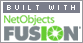Coming to Statistica
in 2022/2023

Zoom tutorials
using Explaineverything app
We a record sessions for you to listen to later

CALL STATISTICA NOW

.

E-mail     anne@statistica.com.au

# Place         Willetton, Western AustraliaSPSS Instructions Mean, Median, Standard Deviation, Variance etc...(these are generated automatically in this command) Confidence Interval other than 95% and Lower and Upper quartiles for one (and two independent samples) One Sample T  test Regression One Sample T  test AnalyzeCompare MeansOne-Sample T Test...  Move the  variable into test variable via the arrow.Put the value of the Null  Hypothesis in as the test value ie  Null  Hypothesis:                m  =  24  Alternative Hypothesis:     m  >  24  Test Value     24  Please make sure you change it from  zero as it will still do the test of significance but it will be wrong unless the value in the Null Hypothesis is zero.   Back to the top Test for  Normality with one (or two independent samples) AnalyzeDescriptive StatisticsExplorePut variable into Dependent list(If 2 independent samples put Type into factor list, and Data into Dependent list) Plots Click Normality with plotsContinue OK Look at Shapiro-Wilk Sig if this is  bigger than 0.05 then the data is normally distributed.   Back to the top Confidence Interval other than 95% and Lower and Upper quartiles for one (and two independent samples) AnalyzeDescriptive StatisticsExplorePut variable into Dependent list (If 2 samples put Type into factor list and Data into Dependent list) Statistics  Change Confidence Interval for mean to new value and tick percentilesContinue OK   Back to the top  Paired  Sample T-testsTransformCompute VariableType the word ‘ Difference’ in the Target VariableThen Group 1 – Group 2Continue Now follow Test of Normality as before but with the variable ‘ Difference’Paired  Sample T-tests AnalyzeCompare MeansPaired-Samples T TestMove the variables into Paired Variable 1 and Variable 2( Note the way the difference was taken back in transform and do the same order here)OK Another method is to use one sample t-test with the differenceOne Sample T testAnalyzeCompare MeansOne-Sample T Test...Move the variable, difference into test variable via the arrow.Put the value of the Null Hypothesis in as the test value e.g.  Back to the top Independent Two Samples T-test In variable viewName one column Data, this should be numericName the other column Type or Group, this should be string,Then in values you can put your letters and labels (you can put words such as with device)  must press add after each one.Please make sure that you put your data in the columns correctly.  One column should have all the data, Second Column should be the groups AnalyzeCompare MeansIndependent-Samples T TestMove the  data variable to Test VariableMove the group variable(Type) to Grouping VariableType(??) will appear,Click Define groupsPut A (1) in Group 1Put B (2) in Group 2  If you want the subtraction A - B, as  SPSS will always take Group One - Group TwoContinue  Back to the top   Regression Put the data into two columns AnalysisRegressionLinearPut the y-value in the dependent (this is the one to be predicted)Put the x-value in the independent SaveResiduals  UnstandardisedPredicted ValueUnstandardisedFor the Regression LineGraphLegacy DialogsInteractiveScatterplotPut the x-value horizontal    Put the y-value verticalFitMethod RegressionFor the Residual plotGraph Legacy DialogsInteractiveScatterplotPut the x-value horizontalPut the sresid verticalFitMethod Mean Mean, Median, Standard Deviation, Variance etc... (these are generated automatically in this command) AnalyzeDescriptive StatisticsExplorePut variable into Dependent list(If 2 independent samples put Type into factor list, and Data into Dependent list) Plots Tick or untick histogram, boxplot stem and leaf plot as required ContinueOK Back to the top[Home] [Parents] [Contact us] [Services] [Mathematics] [Statistics] [School]